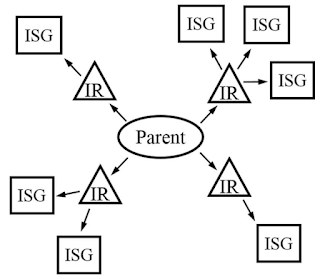Figure. 1. Isotropy subgroup .

This blog contains some key notes for reading the lecture notes by H. Stokes, et al.  on isotropy subgroup. Details about the lecture notes will not be reproduced here and instead we will just put down some key understanding as below,

Two subspaces are equivalent if the basis vectors for each can be set up in such a way that they are associated with the same representation. The reason why the two subspaces are equivalent is that it is actually the linear combination coefficients that appear in front of the basis vectors that determines how the symmetry is lowered. Even though the basis vectors of the two subspaces may be very different, the same linear combination of basis vectors still lead to the same variation of symmetry, e.g., lowering the space group from $$G$$ to its subgroup $$G'$$. This might be the reason why subgroups associated with vectors in parameter space are called isotropy subgroup – atomic distortions in the two equivalent subspaces could be very different in 3D space (i.e., isotropic) but they both lead to the same symmetry lowering from $$G$$ to $$G'$$.

In group theory, there are many ways of separating out group elements into different parts, like cosets. One of the ways is that the separated-out parts themselves can be regarded as a single group element, in which case we say the original group is mapped onto another group the elements of which is actually a group of elements instead of single operations. In such a situation, for sure there should be a certain group of elements that are mapped to the identify element in the new group i.e., all the group elements involved are mapped to the identity element (of the new group). Representation is a certain type of mapping – it maps group elements in the original group to certain matrices in the new group (of matrices which are now the specific form of operations). Then in the context of isotropy subgroup, if a certain isotropy subgroup is mapped to the identity matrix by a certain irreducible representation (IR), that isotropy subgroup is called the kernel of the IR. This further means all vectors in the representation space is invariant under the operation of any elements in that isotropy subgroup (the kernel of the IR).

References